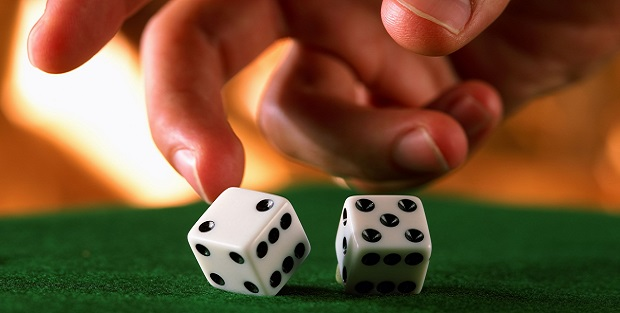# GCSE Maths – ProbabilityAll probabilities are numbers between 0 and 1 – with a probability of 0 signifying that an event is impossible and a probability of 1 signifying that an event must happen.

We will use the terminology that P(A) = the probability that event A happens and N(A) = the number of different ways in which Event A can happen. Providing we can assume that each of the possible outcomes is equally likely then we can define the probability of an event happening as P(A) = N(A) / N(Total) where N(Total) is the total number of possible outcomes.

Example

What is the probability of rolling a six with a fair dice?

There are six possible outcomes – rolling a 1, a 2, a 3, a 4, a 5 or a 6 – so

P(rolling a 6) =N(rolling a 6) / N(different possible outcomes) = 1 / 6

It is often required to work out the probability of a number of events happening. This is done by combining the probabilities of the individual events; how this is done depends on the details of the situation.

Providing the events are mutually exclusive (i.e. only one of the events can happen at any particular occurrence):

·        If we want the probability of any of a number of different events happening at a single time, i.e. A or B, then the individual probabilities are added together.

·        If we want the probability of a series of events happening in sequence, i.e. A and B, then the individual probabilities are multiplied together.

Examples

1) What is the probability of throwing either a 1 or a 6 with a single dice?

P(a 1 or a 6) = P(a 1) + P(a 6) = 1/6 + 1/6 = 2/6

2) You roll two dice, one red and the other blue. What is the probability of throwing a 1 on the red dice and a 6 on the blue dice?

P(a 1 on red and a 6 on blue) = P(a 1 on red) x P(a 6 on blue) = 1/6 x 1/6 = 1/36

If the two dice were not different colours, then a 1 on red + a 6 on blue could not be distinguished from 6 on red and 1 on blue, and so now

P(a 1 and a 6) = P(a 1 on red and a 6 on blue) or (a 6 on red and a 1 on blue) = 1/36 + 1/36 = 1/18

At any stage in a process, the sum of the probabilities of all the possible events that could happen must be 1 (because something must happen!). This has many applications:

·        The probability of an event happening = 1 – the probability that it doesn’t happen.

·        The probability of something happening at least once in a number of attempts = 1 – the probability that it never happens in any of the attempts.

Examples

1) If the probability that your team win their next game is 0.35 and the probability of a draw is 0.2 then what is the probability that they lose?

P(win) + P(draw) + P(lose) = 1, so 0.35 + 0.2 + P(lose) = 1, thus P(lose) = 0.45

2) What is the probability of getting at least one 6 when you throw 4 dice?

P(at least one 6 on four dice) = 1 – P(no 6 on four dice) = 1 – (5/6)4 = 1 –  625/1296 = 671/1296 ≈51.8%

[i.e. there is better than 50:50 odds of getting one 6 on 4 dice]

### Work Towards That 'A' Grade

Achieve the results you want with-written revision resources when you register with iRevise.
Its Free!